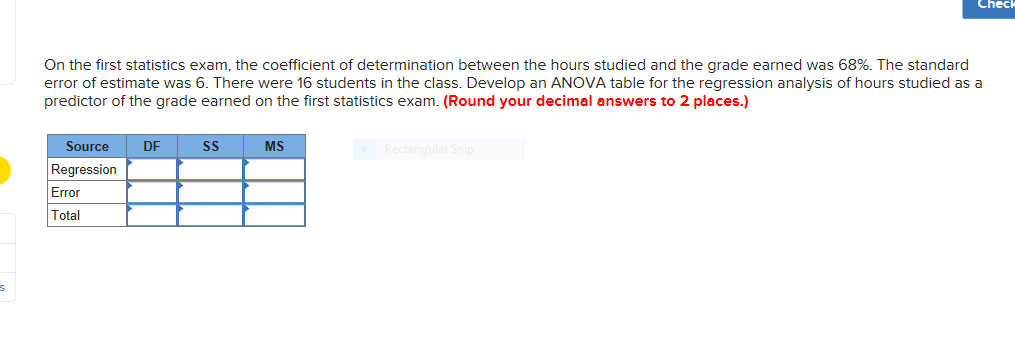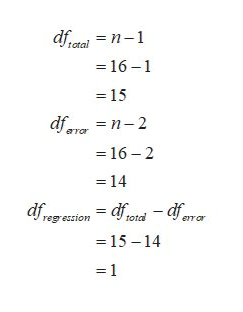# CheckOn the first statistics exam, the coefficient of determination between the hours studied and the grade earned was 68%. The standarderror of estimate was 6. There were 16 students in the class. Develop an ANOVA table for the regression analysis of hours studied as apredictor of the grade earned on the first statistics exam. (Round your decimal answers to 2 places.)Rectangular SniSourceDFSSMSRegressionErrorTotal

Questionhelp_outlineImage TranscriptioncloseCheck On the first statistics exam, the coefficient of determination between the hours studied and the grade earned was 68%. The standard error of estimate was 6. There were 16 students in the class. Develop an ANOVA table for the regression analysis of hours studied as a predictor of the grade earned on the first statistics exam. (Round your decimal answers to 2 places.) Rectangular Sni Source DF SS MS Regression Error Total fullscreen
check_circle

Step 1

The provided information is,

Step 2

The degrees of freedom can be obtained as:help_outlineImage Transcriptionclosedfataln-1 total -16-1 -15 dfern-2 aror -16-2 14 = dfdfrar dfres total regression eror -15-14 - 1 fullscreen
Step 3

The SSE can be ob...

### Want to see the full answer?

See Solution

#### Want to see this answer and more?

Solutions are written by subject experts who are available 24/7. Questions are typically answered within 1 hour.*

See Solution
*Response times may vary by subject and question.
Tagged in

### Statistics